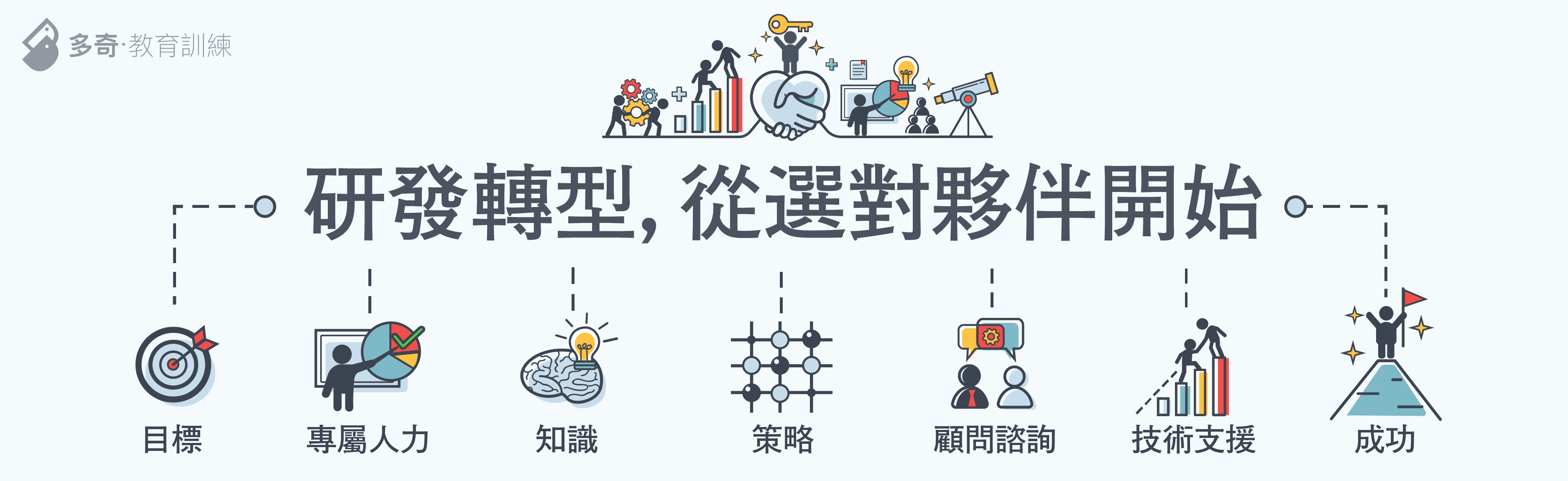分享幾個 LINQ to SQL 執行各種 Join 查詢的技巧 | The Will Will Web# The Will Will Web

### 記載著 Will 在網路世界的學習心得與技術分享```from c in Categories
from o in c.Products
select new { c.CategoryName, o.ProductID, o.ProductName }```

```SELECT [t0].[CategoryName], [t1].[ProductID], [t1].[ProductName]
FROM [Categories] AS [t0], [Products] AS [t1]
WHERE [t1].[CategoryID] = [t0].[CategoryID]```

```from c in Categories
join o in Products on c.CategoryID equals o.CategoryID
select new { c.CategoryName, o.ProductID, o.ProductName }```

```SELECT [t0].[CategoryName], [t1].[ProductID], [t1].[ProductName]
FROM [Categories] AS [t0]
INNER JOIN [Products] AS [t1] ON ([t0].[CategoryID]) = [t1].[CategoryID]```

```from c in Categories
join o in Products on c.CategoryID equals o.CategoryID
select new { o.Category.CategoryName ,o.ProductID, o.ProductName }```

```SELECT [t2].[CategoryName], [t0].[ProductID], [t0].[ProductName]
FROM [Products] AS [t0]
INNER JOIN [Categories] AS [t1] ON [t0].[CategoryID] = ([t1].[CategoryID])
LEFT OUTER JOIN [Categories] AS [t2] ON [t2].[CategoryID] = [t0].[CategoryID]```

```from c in Categories
join o in Products on c.CategoryID equals o.CategoryID
select new { c.CategoryName, o.ProductID, o.ProductName }```

```SELECT [t0].[CategoryName], [t1].[ProductID], [t1].[ProductName]
FROM [Categories] AS [t0]
INNER JOIN [Products] AS [t1] ON ([t0].[CategoryID]) = [t1].[CategoryID]```

```from o in Products
select new { o.Category.CategoryName, o.ProductID, o.ProductName }```

```SELECT [t1].[CategoryName], [t0].[ProductID], [t0].[ProductName]
FROM [Products] AS [t0]
LEFT OUTER JOIN [Categories] AS [t1] ON [t1].[CategoryID] = [t0].[CategoryID]```

```from c in Categories
join o in Products on c.CategoryID equals o.CategoryID
select new { c.CategoryName, o.ProductName }```

```from c in Categories
join o in Products on c.CategoryID equals o.CategoryID into ps
from o in ps.DefaultIfEmpty()
select new { c.CategoryName, o.ProductName }```

```SELECT [t0].[CategoryName], [t1].[ProductName] AS [ProductName]
FROM [Categories] AS [t0]
LEFT OUTER JOIN [Products] AS [t1] ON ([t0].[CategoryID]) = [t1].[CategoryID]```